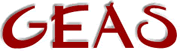[ < ] [ > ] [ << ] [ Up ] [ >> ] [Top] [Contents] [Index] [ ? ]

#### 1.2.5.2 Expressions

An expression is an instruction or set of instructions that results in a value of some kind. Take `+`, for example. It uses two other expressions to make up a result. A variable is an expression since it yields its contents as a result. The combination of the following two expressions and an operator is a valid expression: `a + b`, `a` and `b` being variables (expressions) and `+` being the operator used on them. `a = b + c;` is a full statement ending in a `;`.

Function calls are valid expressions. They are written simply as the name followed by a set of matched parentheses with the arguments that the functions uses listed inside. Take the simple function `max()` for example, that returns the max of the two arguments. To determine the maximum of `4` and `10`, you would write `max(4, 10)` as the expression. Naturally the result must be either stored or used.

This document was generated by Ronny Wikh on July, 8 2003 using texi2html